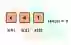# Golang Len (String Length)

Use the len built-in function to get string lengths. Len supports arrays, slices and maps.

Len. This string has 3 runes. It spells "dog." No counting needs to be done to measure its length. The len built-in returns a stored length value.Len details. In this language, we use built-in methods like len on strings, arrays, slices and maps. This may make code clearer—simpler to understand.

String length. We test len() on a string. We assign a variable named "length" to the result of the len function. This returns the count of characters.
Loop: We can loop over the characters in a string by accessing its length. We use all indexes from 0 to the len minus one (inclusive).Golang program that uses len to get string length package main import "fmt" func main() { value := "cat" // Take length of string with len. length := len(value) fmt.Println(length) // Loop over the string with len. for i := 0; i < len(value); i++ { fmt.Println(string(value[i])) } } Output 3 c a t

Slice length. A slice too can be the argument to len(). This returns the number of elements (of any value) in the slice. Here we test len on a 4-element int slice.
Append: We call the append() built-in to add a fifth element to the slice. The len() method then returns 5.
Golang program that uses len, append on slice package main import "fmt" func main() { slice := []int{10, 20, 30, 40} // Get length of slice with len. length := len(slice) fmt.Println(slice) fmt.Println(length) // Append an element, and take the length again. slice = append(slice, 50) fmt.Println(slice) fmt.Println(len(slice)) } Output [10 20 30 40] 4 [10 20 30 40 50] 5

Nil. In Go, a slice may be nil—this is a nonexistent slice. The len of a nil slice is 0. No panic results from taking the len of a nil thing.
Golang program that uses len on nil slice package main import "fmt" func main() { // A slice with len of 3. value := []int{10, 20, 30} fmt.Println(len(value)) // A nil slice has a len of 0. value = nil fmt.Println(len(value)) } Output 3 0

Performance. The performance of len does not depend on the length of the string. Its time is constant. This is achieved in languages by storing the string's length as a field on the object.
So: Len is fast. On my system it can be used 10 million times on a string of any length in less than 10 ms.
Version 1: This version of the code uses the len built-in on a short string of just 3 characters.
Version 2: This version uses len on a longer string of 65 characters. It shows that len returns in constant time.
Golang program that times string len package main import ( "fmt" "time" ) func main() { // Length is 3. string1 := "cat" // Length is 13 times 5 which is 65. string2 := "cat0123456789" + "cat0123456789" + "cat0123456789" + "cat0123456789" + "cat0123456789" t0 := time.Now() // Version 1: length of short string. for i := 0; i < 10000000; i++ { result := len(string1) if result != 3 { return } } t1 := time.Now() // Version 2: length of long string. for i := 0; i < 10000000; i++ { result := len(string2) if result != 65 { return } } t2 := time.Now() // Print results. fmt.Println(t1.Sub(t0)) fmt.Println(t2.Sub(t1)) } Output 7.0049ms len( 3 character string) 7.0189ms len(65 character string)

A summary. Len discovers lengths of things. In an array, the length is part of the type itself. But for strings and slices, a stored value (maintained by the runtime) is returned.

Home

© 2007-2020 sam allen. send bug reports to info@dotnetperls.com.